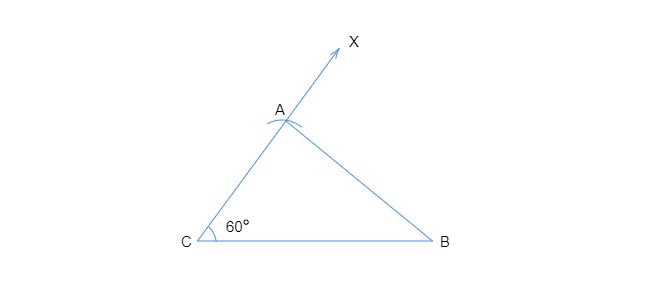# Construct $√ҐЋЖвА†ABC$ with $BC = 7.5\ cm$, $AC = 5\ cm$ and $\angle C = 60^{\circ}$.

To do: To construct $∆ABC$ with $BC = 7.5\ cm$, $AC = 5\ cm$ and $m \angle C = 60^{\circ}$.

Steps of construction :
• Let us draw a line segment $BC$ with the length of $7.5\ cm$.
• From the point $C$, let us draw a ray $CX$ making an angle of $60^{\circ}$ with $BC$ such that $\angle ACB=60^{\circ}$.
• Assuming $C$ as the center, let us draw an arc of radius $5\ cm$ that cuts $CX$ at the point $A$.
• Now let us join $AB$ to get the required triangle.$\triangle ABC$  is the required triangle.

Hence constructed!

Updated on: 10-Oct-2022

32 Views